GRE Subject Test: Math : Fundamental Theorem of Algebra

Example Questions

Example Question #1 : Fundamental Theorem Of Algebra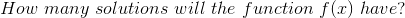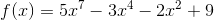Explanation:

The corollary to the Fundamental Theorem of Algebra states that for any polynomial the number of solutions will match the degree of the function.

The degree of a function is determined by the highest exponent for x, which in this case is 7.

This means that there will be 7 solutions total for the below function.This means that max number of REAL solutions would be 7, but the total number of solutions, real, repeated or irrational will total 7.

Example Question #12 : Polynomials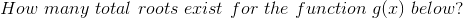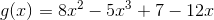Explanation:

In order to determine the correct answer, we must first change the function to be in standard form. A function in standard form begins with the largest exponent then decreases from there.

We must change:to become: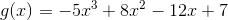Once we have established standard form, we can now see that this is a degree 3 polynomial, which means that it will have 3 roots or solutions.

Example Question #21 : Polynomials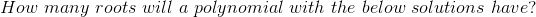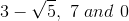Explanation:

If a complex or imaginary root exists, its' complex conjugate must also exist as a root.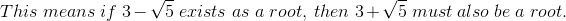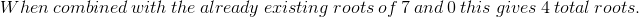Example Question #22 : Polynomials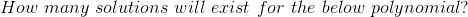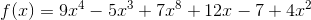Explanation:

Based upon the corollary to the Fundamental Theorem of Algebra, the degree of a function determines the number of solutions/zeros/roots etc. that exist. They may be real, repeated, imaginary or irrational.

In this case, we must first change the function to be in standard form before determining the degree. Standard form means that the largest exponent goes first and the terms are organized by decreasing exponent.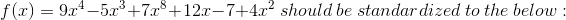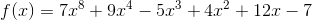Now that the polynomial is in standard form, we see that the degree is 8.

There exists 8 total solutions/roots/zeros for this polynomial.

All GRE Subject Test: Math Resources